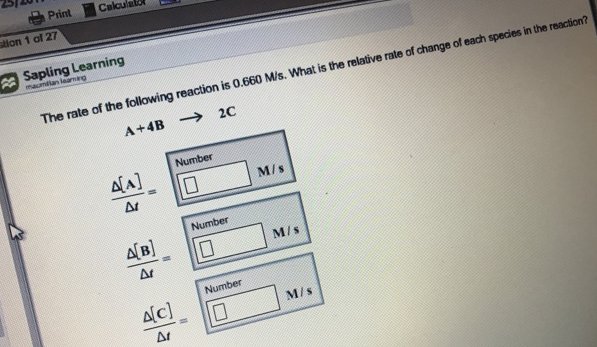# Problem: The rate of the following reaction is 0.660 M/s. What is the relative rate of change of each species in the reaction? A + 4B → 2C

###### FREE Expert Solution###### Problem Details

The rate of the following reaction is 0.660 M/s. What is the relative rate of change of each species in the reaction?

A + 4B → 2C